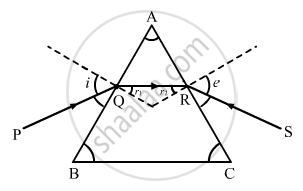Share

# Figure Shows a Ray of Light Passing Through a Prism. If the Refracted Ray Qr is Parallel to the Base Bc, Show that (I) R1 = R2 = A/2 and (Ii) Angle of Minimum Deviation, Dm = 2i − A. - Physics

ConceptDispersion by a Prism

#### Question

Figure shows a ray of light passing through a prism. If the refracted ray QR is parallel to the base BC, show that (i) r1 = r2 = A/2 and (ii) angle of minimum deviation, Dm = 2i − A.#### Solution

(i) When QR is parallel to the base BC, we have: i = e

$\Rightarrow r_1 = r_2 = r$

We know that

$r_1 + r_2 = A$

$\Rightarrow r + r = A$

$\therefore r = A/2$

(ii) Also, we have:
A + D= i + e
Substituting, D = Dand e = i
A + Dm= i + i
∴ Dm=2i - A

Is there an error in this question or solution?

#### Video TutorialsVIEW ALL 

Solution Figure Shows a Ray of Light Passing Through a Prism. If the Refracted Ray Qr is Parallel to the Base Bc, Show that (I) R1 = R2 = A/2 and (Ii) Angle of Minimum Deviation, Dm = 2i − A. Concept: Dispersion by a Prism.
S